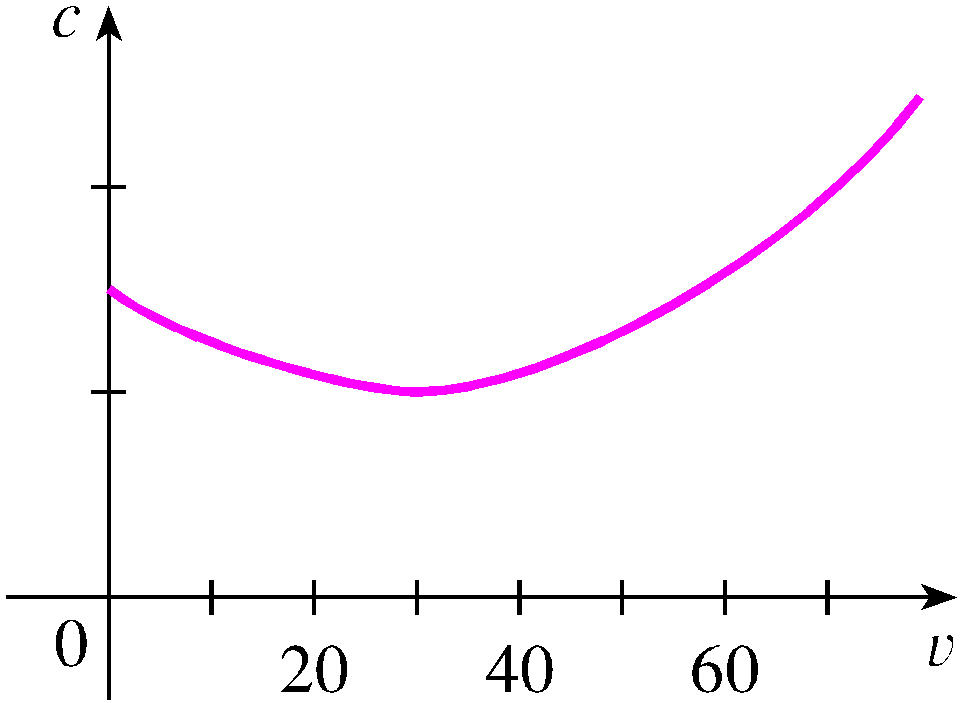The graph shows the fuel consumption c of a car (measured in gallons per hour) as a function of the speed v of the car. At very low speeds the engine runs inefficiently, so initially c decreases as the speed increases. But at high speeds the fuel consumption increases. You can see that c(v) is minimized for this car when v is about 30 mi/h. However, for fuel efficiency, what must be minimized is not the consumption in gallons per hour but rather the fuel consumption in gallons per mile. Let's call this consumption G. Using the graph, estimate the speed at which G has its minimum value.mi/h